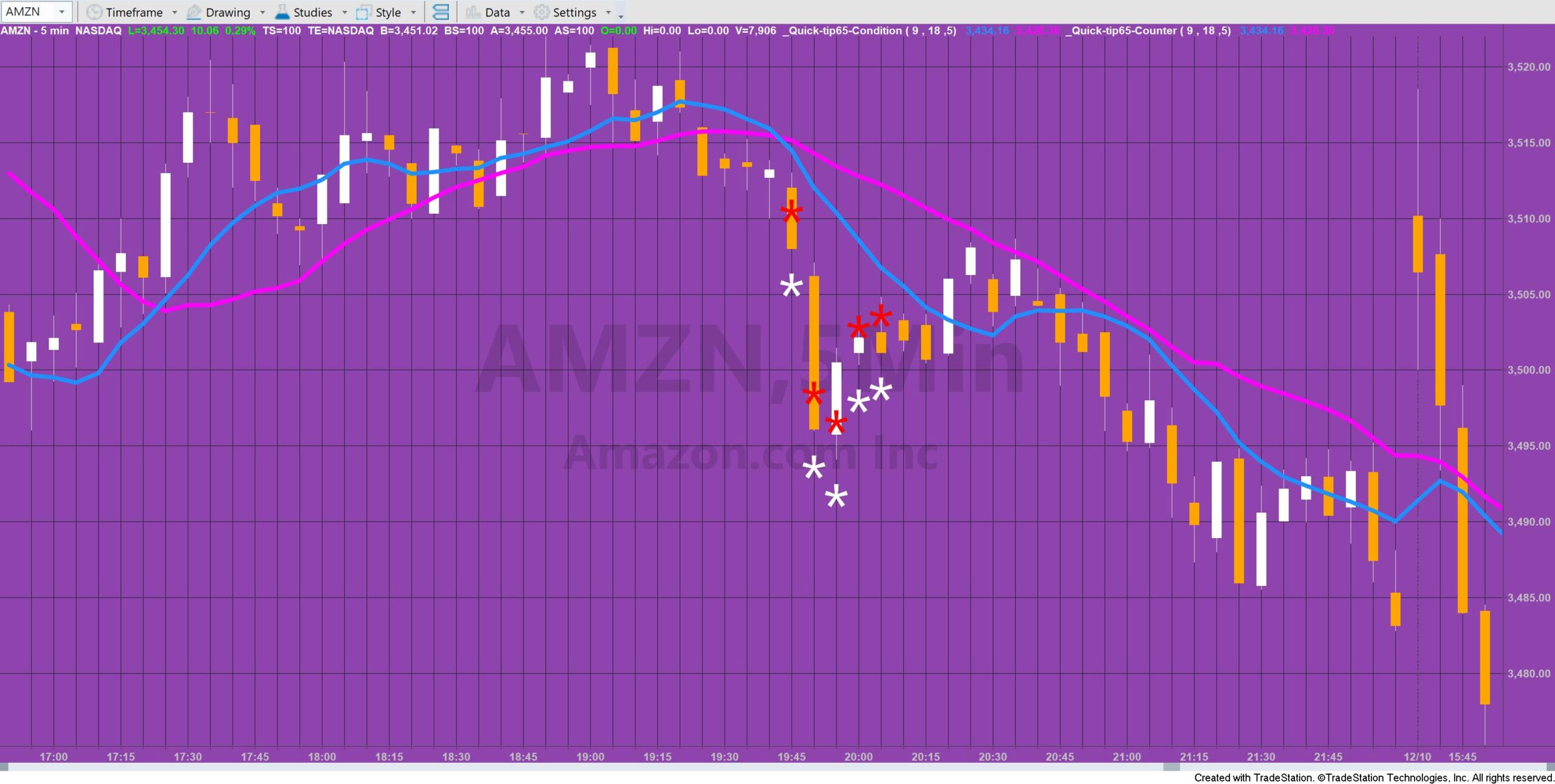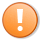# Quick-tip 65 | Number of bars after cross

Quick-tip 65 demonstrates two simple ways of finding and showing when a condition on the chart has occurred within a user input number of bars.

The quick-tip shows two ways that this can be programmed. The condition used to facilitate the demonstration is the cross of a fast moving average over a slow moving average.

### Using a counter

This first technique is used in the TradeStation indicator: _Quick-tip65-Counter. In this program a counter indicator is incremented by one every bar.  When the fast moving average crosses over a slow moving average the counter is reset to zero. The program draws an asterisk when the value of the counter is less than a user input value, i.e. when the cross had occurred less that that number of bars ago.

### Setting a variable

In the second technique (_Quick-tip65-Condition) a variable is set to a value of 1 every time the fast moving average crosses over a slow moving average, otherwise is is set to zero. The summation function is used to check whether the sum of this variable over the user input number of bars is greater than zero. If so an asterisk is drawn on the chart.

In this example, the asterisks are displayed vertically.The example indicator(s) applied to a chart

### Video explanation of quick-tip 65This content is for members only.